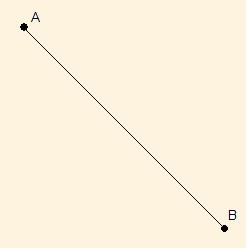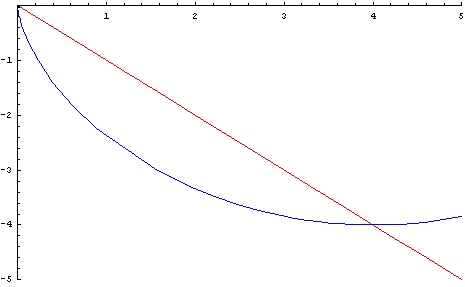# Brachistochrone

 The discussion begins with a simple question, which has a simple answer and a not-so-simple answer. "What is the shortest distance between 2 points?". Simple you might say. A straight line. Correct. The shortest geometrical distance is a straight line. Now lets add a new dimension to our question - time - and ask the question in a different manner. "What is the shortest distance between 2 points?"! Isn't it the same question we asked before? Yes. No kidding. But since we want to factor in time, this question now takes on a different form. The "shortest distance between 2 points A & B" can now also be understood as "fastest time taken to travel from A to B.". Now, is the answer a straight line? No! Let's see what it is. Imagine you have been called in at a water park to design a water slide that starts from a vantage point (say A) and ends in a pool (point B). To add the element of thrill to the ride, you are asked to build the slide so that it takes the person from point A down to point B in fastest possible time. What shape would you build it? Straight down from A to B?Similar question was first posed by Johann Bernoulli in 1696 to contemporary mathematicians. After a period of several months, he received solutions from 5 mathematicians. His brother Jacob Bernoulli, L'Hospital, Tschirnhaus (inventor of porcelain), Leibniz and Newton. So the answer we seek is not a straight line, as we can see from the animation below. The path of fastest descent is not a straight line!The "shortest distance", or the path of fastest descent is a curve, called cycloid. Interestingly, a cycloid can be formed by fixing a point on a wheel, and tracing the path followed by the point as the wheel rolls. This is illustrated below.So, the shape of your water slide is going to be a cycloid. The next time you are asked about the shortest distance between 2 points, surprise your audience with a cycloid! So, why is it called a brachistochrone? In Greek, brachistos stands for shortest and chronos stands for time. Hence the name. The brachistochrone has an interesting history on account of the rivalry between the Bernoulli brothers, the way Johann Bernoulli tried to intimidate contemporary mathematicians with difficult problems, and Newton's persistence in solving the brachistochrone problem. To read more about the history, visit Brachistochrone
 Quick Comment : * Name Email ( Your email id is safe with us! ) * Comments * Type the number of characters in the word "HUMAN"